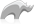##### [ timer ]

Method

A simple timer to time blocks of code.

Returns an array containing the total time taken for all iterations (micros_total) and average time taken per iteration (micros_average).

• Syntax
``timer(1) => { }``
##### Examples
• Beginner
• Intermediate

Time a single iteration.

Code

``timer(1) => { sleep(5) }``

Result

`array((micros = 5184.000000), (micros_average = 5184.000000))`

To use the time taken on a block of code you can set the timer section to a variable. Then you can get the results like you would normally.

Code

``````	var('timeTaken') = timer(1) => {

loop(1000000) => {

}

}

'That section of code took ' + \$timeTaken->first->second * .000001 + ' seconds.';``````

Result

`That section of code took 0.489982 seconds.`

Time multiple iterations.

Code

``timer(1000) => { sleep(5) }``

Result

`array((micros = 5276669.000000), (micros_average = 5276.669000))`

##### Resources
• Tips

When testing small chunks of code the more iterations you use the more acturate the average will be. 10,000+ is good for tiny portions of code.Please note that periodically LassoSoft will go through the notes and may incorporate information from them into the documentation. Any submission here gives LassoSoft a non-exclusive license and will be made available in various formats to the Lasso community.